Magnetic Field of Current Loop

 Examining the direction of the magnetic field produced by a current-carrying segment of wire shows that all parts of the loop contribute magnetic field in the same direction inside the loop.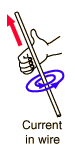Electric current in a circular loop creates a magnetic field which is more concentrated in the center of the loop than outside the loop. Stacking multiple loops concentrates the field even more into what is called a solenoid.Field at center Field on axis
Index

Magnetic field concepts

Currents as magnetic sources

 HyperPhysics***** Electricity and Magnetism R Nave
Go Back

Field at Center of Current LoopThe form of the magnetic field from a current element in the Biot-Savart law becomes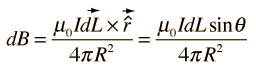which in this case simplifies greatly because the angle =90 ° for all points along the path and the distance to the field point is constant. The integral becomes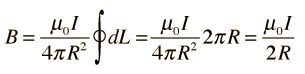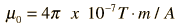For a current I = Amperes and
 loop radius R = m, the magnetic field at the center of the loop is
 B = x 10^ Tesla = Gauss.

At a distance z = m out along the centerline of the loop, the axial magnetic field is

 B = x 10^ Tesla = Gauss.

The current used in the calculation above is the total current, so for a coil of N turns, the current used is Ni where i is the current supplied to the coil.

The Earth's magnetic field at the surface is about 0.5 Gauss.

Discussion of current loop
Index

Magnetic field concepts

Currents as magnetic sources

 HyperPhysics***** Electricity and Magnetism R Nave
Go Back

Field on Axis of Current Loop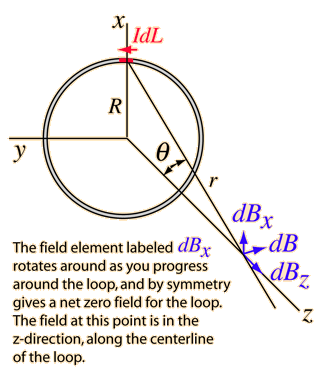Show geometric details

The application of the Biot-Savart law on the centerline of a current loop involves integrating the z-component.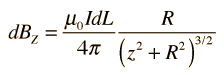The symmetry is such that all the terms in this element are constant except the distance element dL , which when integrated just gives the circumference of the circle. The magnetic field is then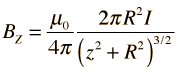Calculation

Discussion of current loop
Index

Magnetic field concepts

Currents as magnetic sources

 HyperPhysics***** Electricity and Magnetism R Nave
Go Back

Geometry: Field on Axis of Current LoopDiscussion of current loop
Index

Magnetic field concepts

Currents as magnetic sources

 HyperPhysics***** Electricity and Magnetism R Nave
Go Back